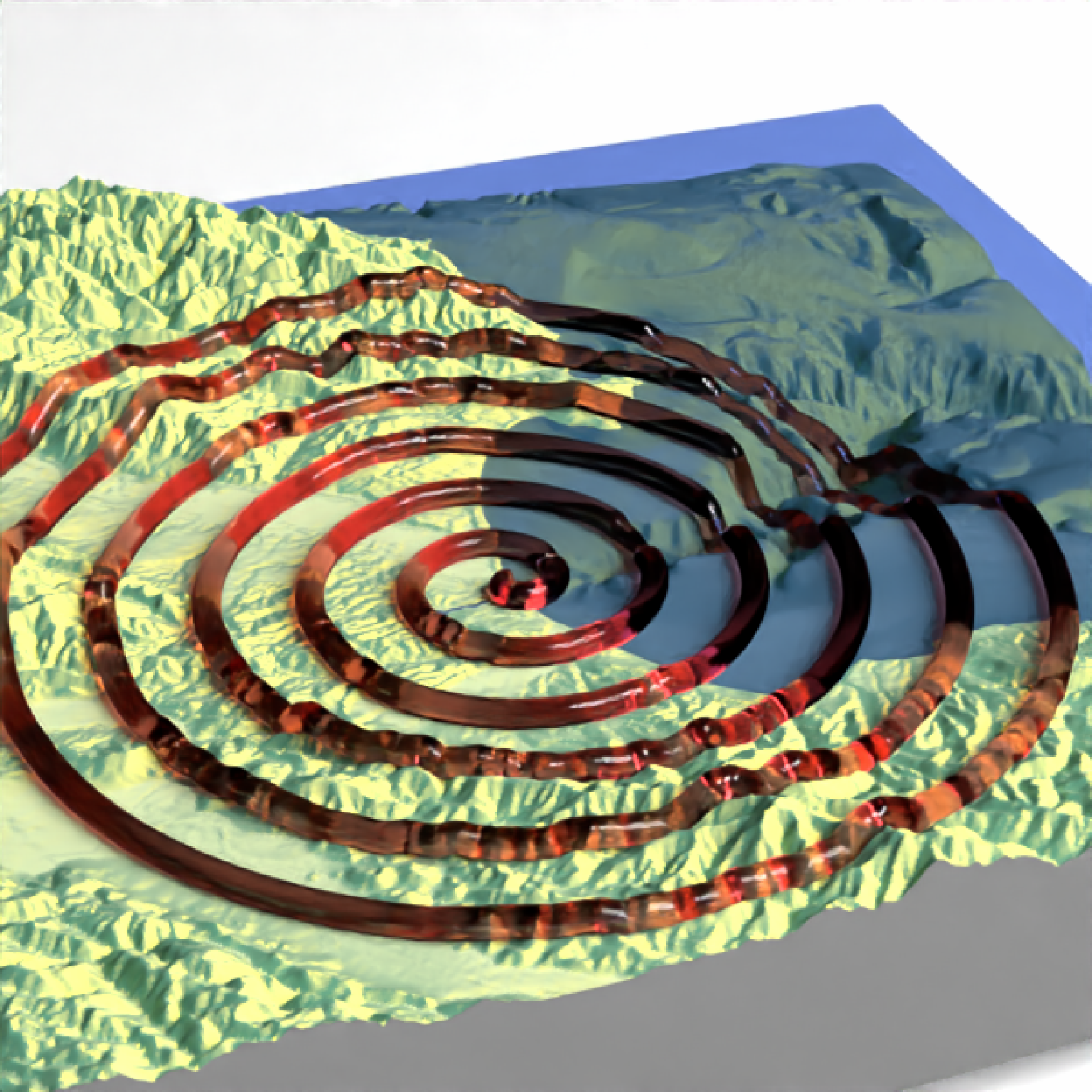Adds a 3D path to the current scene, using latitude/longitude or coordinates in the reference system defined by the extent object. If no altitude is provided, the path will be elevated a constant offset above the heightmap. If the path goes off the edge, the nearest height on the heightmap will be used.

render_path(
lat,
long = NULL,
altitude = NULL,
groups = NULL,
extent = NULL,
zscale = 1,
heightmap = NULL,
resample_evenly = FALSE,
resample_n = 360,
reorder = FALSE,
reorder_first_index = 1,
reorder_duplicate_tolerance = 0.1,
reorder_merge_tolerance = 1,
simplify_tolerance = 0,
linewidth = 3,
color = "black",
antialias = FALSE,
offset = 5,
clear_previous = FALSE,
return_coords = FALSE,
tag = "path3d"
)

## Arguments

lat

Vector of latitudes (or other coordinate in the same coordinate reference system as extent). Can also be an sf or SpatialLineDataFrame object.

long

Default NULL. Vector of longitudes (or other coordinate in the same coordinate reference system as extent). Ignored if lat is an sf or SpatialLineDataFrame object.

altitude

Default NULL. Elevation of each point, in units of the elevation matrix (scaled by zscale). If left NULL, this will be just the elevation value at ths surface, offset by offset. If a single value, all data will be rendered at that altitude.

groups

Default NULL. Integer vector specifying the grouping of each lat/long path segment, if lat/long are specified as numeric vectors (as opposed to sf or SpatialLineDataFrame objects, where this information is built-in to the object).

extent

Either an object representing the spatial extent of the 3D scene (either from the raster, terra, sf, or sp packages), a length-4 numeric vector specifying c("xmin", "xmax","ymin","ymax"), or the spatial object (from the previously aforementioned packages) which will be automatically converted to an extent object.

zscale

Default 1. The ratio between the x and y spacing (which are assumed to be equal) and the z axis in the original heightmap.

heightmap

Default NULL. Pass this if not including an altitude argument, or if no extent passed. A two-dimensional matrix, where each entry in the matrix is the elevation at that point. All points are assumed to be evenly spaced.

resample_evenly

Default FALSE. If TRUE, this will re-sample the path evenly from beginning to end, which can help vastly reduce the number of points used to draw it (which can improve the performance of render_highquality() and render_snapshot(software_render = TRUE)). This function works only if reorder = TRUE, or if the sf object is already ordered from beginning to end.

resample_n

Default 360. Number of breaks in which to evenly resample the line if resample_evenly = TRUE.

reorder

Default FALSE. If TRUE, this will attempt to re-order the rows within an sf object with multiple paths to be one continuous, end-to-end path. This happens in two steps: merging duplicate paths that have end points that match with another object (within reorder_duplicate_tolerance distance), and then merges them (within reorder_merge_tolerance distance) to form a continuous path.

reorder_first_index

Default 1. The index (row) of the sf object in which to begin the reordering process. This merges and reorders paths within reorder_merge_tolerance distance until it cannot merge any more, and then repeats the process in the opposite direction.

reorder_duplicate_tolerance

Default 0.1. Lines that have start and end points (does not matter which) within this tolerance that match a line already processed (order determined by reorder_first_index) will be discarded.

reorder_merge_tolerance

Default 1. Lines that have start points that are within this distance to a previously processed line's end point (order determined by reorder_first_index) will be reordered within the sf object to form a continuous, end-to-end path.

simplify_tolerance

Default 0 (no simplification). If greater than zero, simplifies the path to the tolerance specified. This happens after the data has been merged if reorder = TRUE. If the input data is specified with long-lat coordinates and sf_use_s2() returns TRUE, then the value of simplify_tolerance must be specified in meters.

linewidth

Default 3. The line width.

color

Default black. Color of the line.

antialias

Default FALSE. If TRUE, the line with be have anti-aliasing applied. NOTE: anti-aliasing can cause some unpredictable behavior with transparent surfaces.

offset

Default 5. Offset of the track from the surface, if altitude = NULL.

clear_previous

Default FALSE. If TRUE, it will clear all existing paths.

return_coords

Default FALSE. If TRUE, this will return the internal rayshader coordinates of the path, instead of plotting the line.

tag

Default "path3d". The rgl tag to use when adding the path to the scene.

## Examples

if(run_documentation()) {
#Starting at Moss Landing in Monterey Bay, we are going to simulate a flight of a bird going
#out to sea and diving for food.

#First, create simulated lat/long data
set.seed(2009)
moss_landing_coord = c(36.806807, -121.793332)
x_vel_out = -0.001 + rnorm(1000)[1:300]/1000
y_vel_out = rnorm(1000)[1:300]/200
z_out = c(seq(0,2000,length.out = 180), seq(2000,0,length.out=10),
seq(0,2000,length.out = 100), seq(2000,0,length.out=10))

bird_track_lat = list()
bird_track_long = list()
bird_track_lat[] = moss_landing_coord
bird_track_long[] = moss_landing_coord
for(i in 2:300) {
bird_track_lat[[i]] = bird_track_lat[[i-1]] + y_vel_out[i]
bird_track_long[[i]] = bird_track_long[[i-1]] + x_vel_out[i]
}

#Render the 3D map
montereybay %>%
plot_3d(montereybay,zscale=50,water=TRUE,
theta=210,  phi=22, zoom=0.20, fov=55)

#Pass in the extent of the underlying raster (stored in an attribute for the montereybay
#dataset) and the latitudes, longitudes, and altitudes of the track.
render_path(extent = attr(montereybay,"extent"),
lat = unlist(bird_track_lat), long = unlist(bird_track_long),
altitude = z_out, zscale=50,color="white", antialias=TRUE)
render_snapshot()
}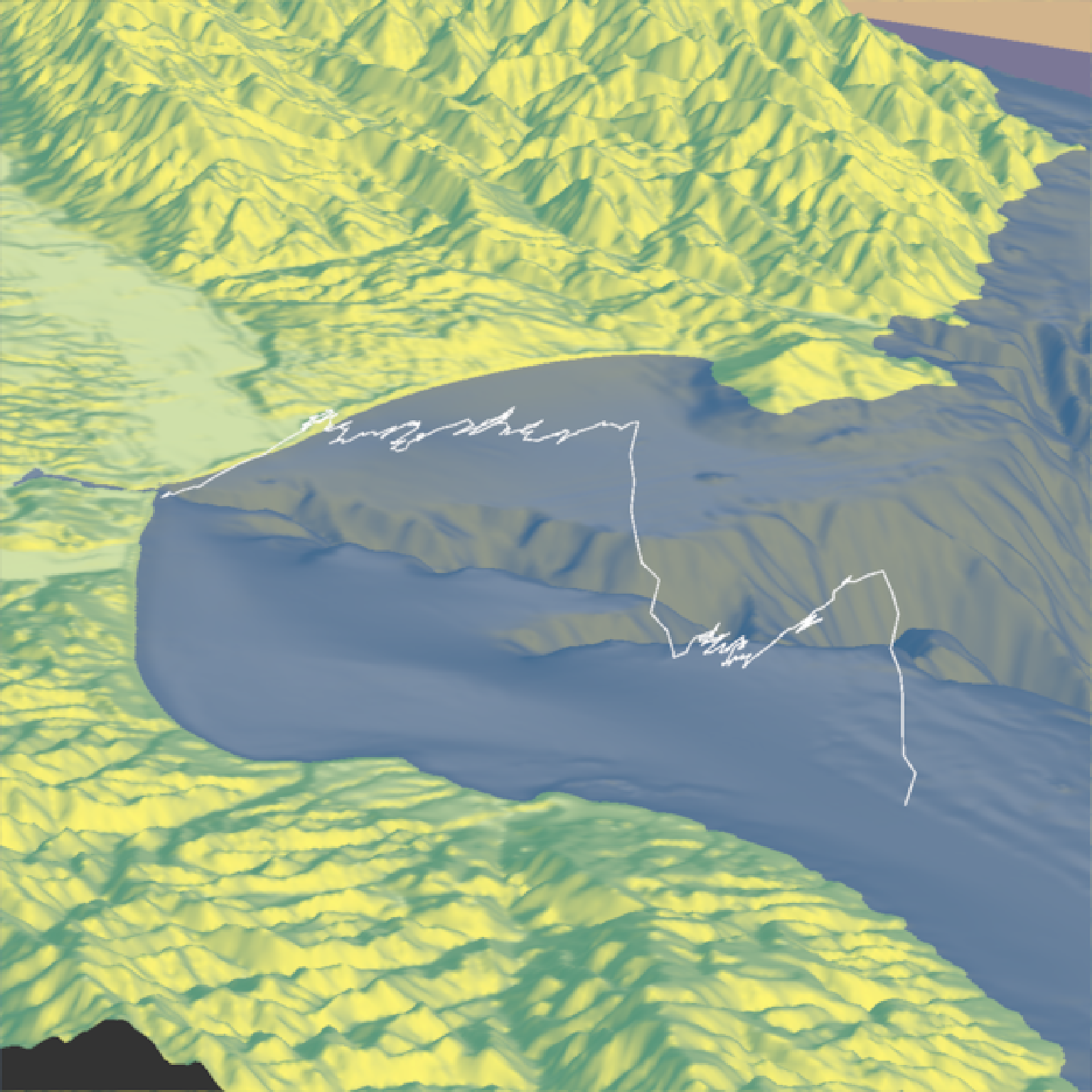if(run_documentation()) {
#We'll set the altitude to right above the water to give the tracks a "shadow".
render_path(extent = attr(montereybay,"extent"),
lat = unlist(bird_track_lat), long = unlist(bird_track_long),
altitude = 10, zscale=50, color="black", antialias=TRUE)
render_camera(theta=30,phi=35,zoom=0.45,fov=70)
render_snapshot()
}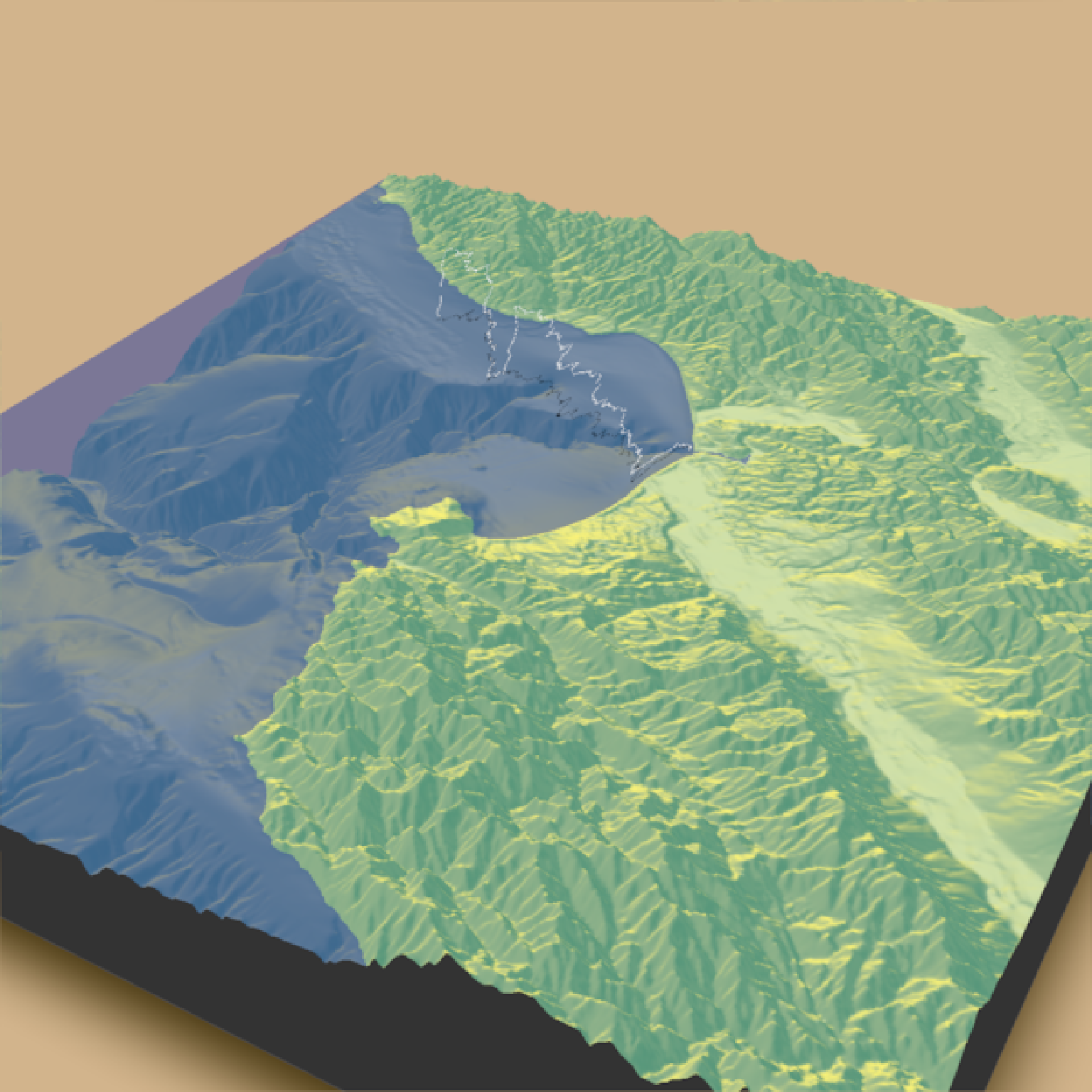if(run_documentation()) {
#Remove the path:
render_path(clear_previous=TRUE)

#Finally, we can also plot just GPS coordinates offset from the surface by leaving altitude NULL
# Here we plot a spiral of values surrounding Moss Landing. This requires the original heightmap.

t = seq(0,2*pi,length.out=1000)
circle_coords_lat = moss_landing_coord + 0.5 * t/8 * sin(t*6)
circle_coords_long = moss_landing_coord + 0.5 * t/8 *  cos(t*6)
render_path(extent = attr(montereybay,"extent"), heightmap = montereybay,
lat = unlist(circle_coords_lat), long = unlist(circle_coords_long),
zscale=50, color="red", antialias=TRUE,offset=100, linewidth=5)
render_camera(theta = 160, phi=33, zoom=0.4, fov=55)
render_snapshot()
}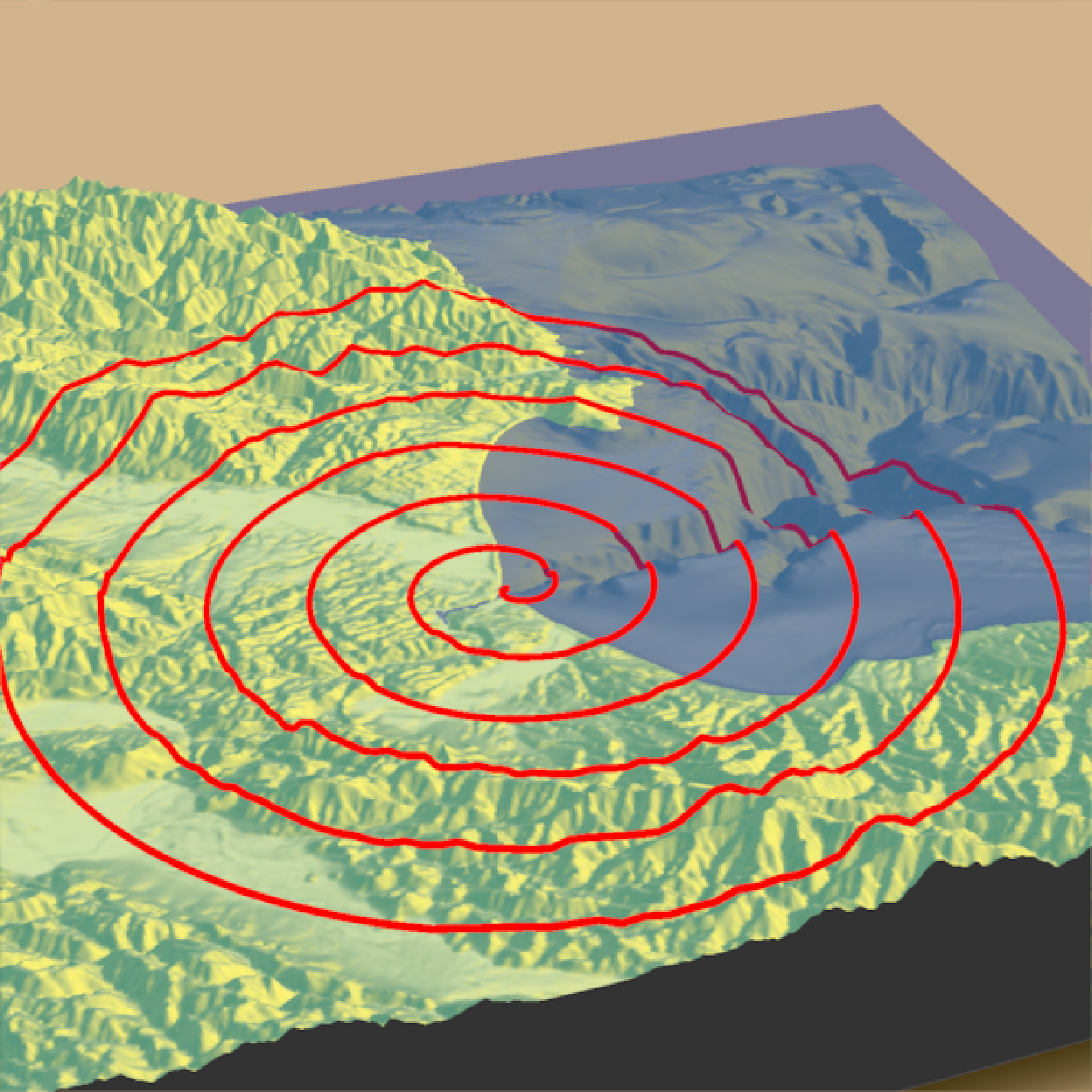if(run_documentation()) {
#And all of these work with render_highquality()
sample_method = "sobol_blue", samples = 128)
}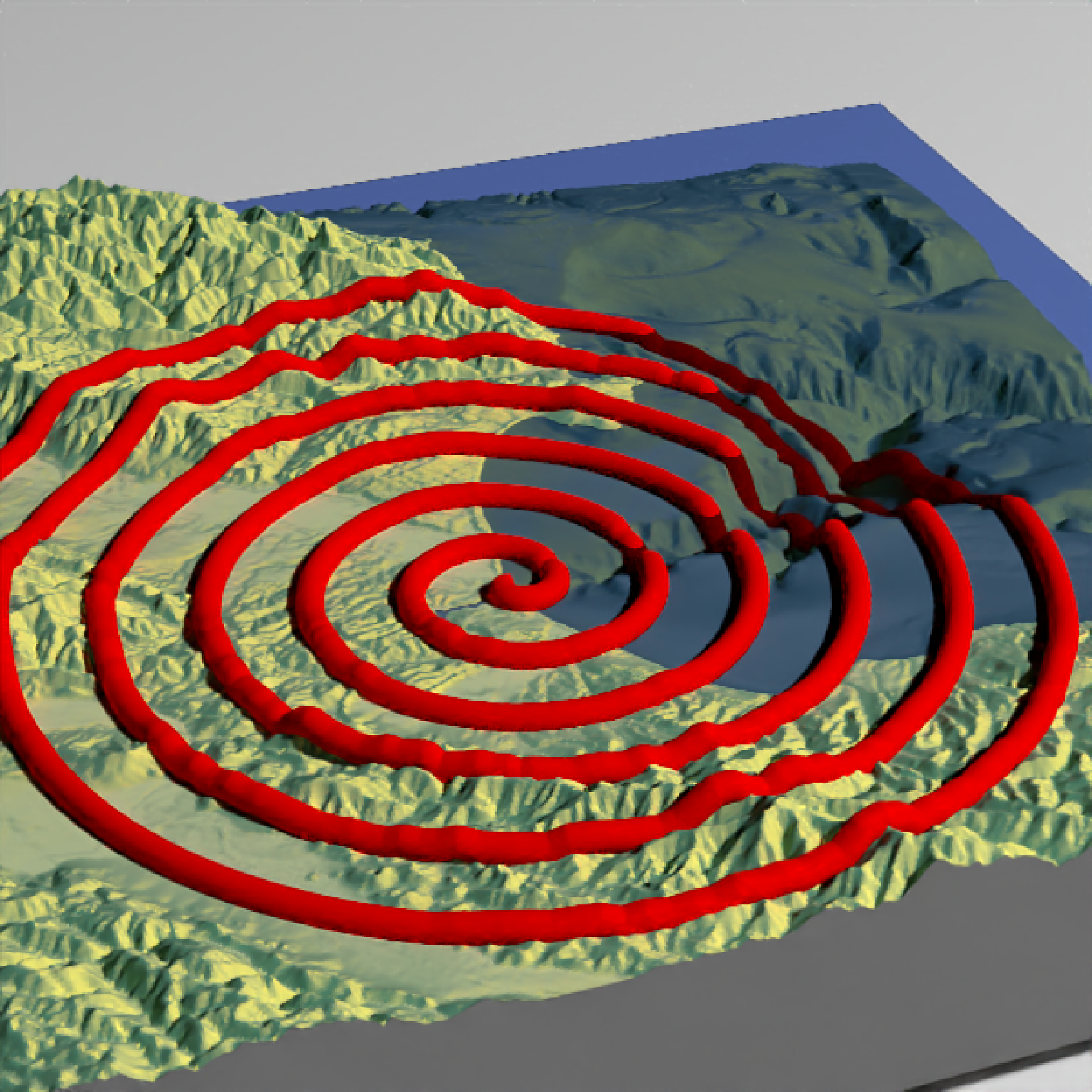if(run_documentation()) {
#We can also change the material of the objects by setting the point_material and
#point_material_args arguments in render_highquality()
sample_method = "sobol_blue", samples = 128,
path_material = rayrender::glossy,
path_material_args = list(gloss = 0.5, reflectance = 0.2))
}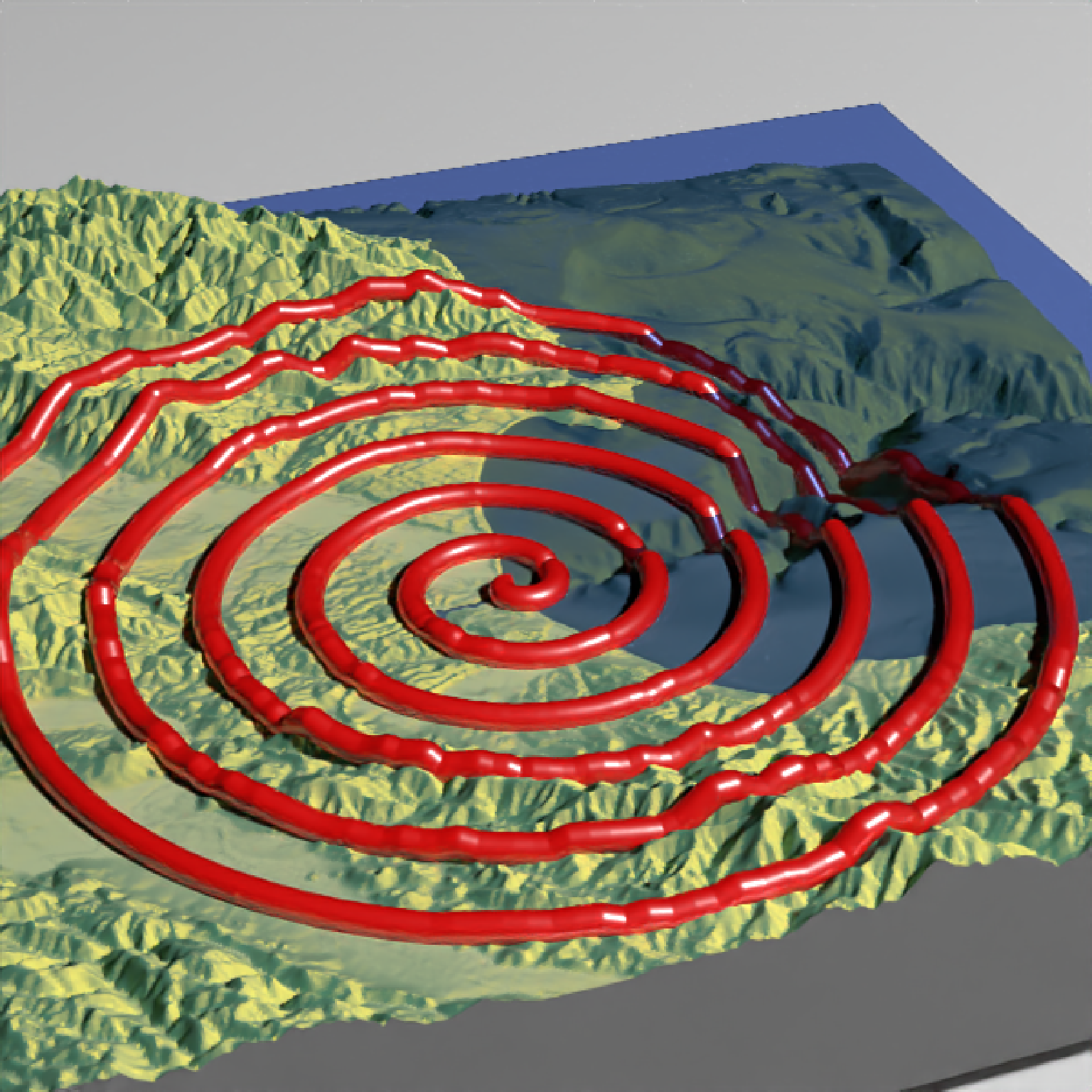if(run_documentation()) {
#For transmissive materials (like dielectric), we should specify that the path
#should be rendered with an extruded path. We'll use the attenuation argument in
#the dielectric function to specify a realistic glass color.
render_path(extent = attr(montereybay,"extent"), heightmap = montereybay, clear_previous = TRUE,
lat = unlist(circle_coords_lat), long = unlist(circle_coords_long),
zscale=50, color="white", offset=200, linewidth=5)